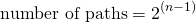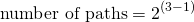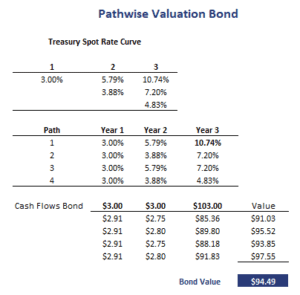## Pathwise Valuation Bond

Pathwise valuation of a bond is a method to price a bond. The pathwise approach is mathematically identical to valuing a bond using the backward induction method. In particular, an interest rate tree with n periods means that there are 2^(n-1) unique paths. We can calculate the bond’s price under each path and calculate the value of the bond as the average of these prices.

On this page, we discuss the pathwise valuation method in in detail and implement a pathwise bond valuation Excel example. The spreadsheet used to perform the calculations is available at the bottom of the page.

## Pathwise valuation bond formula

The first thing we should figure out when we want to apply pathwise valuation is the number of paths. The pathwise valuation number of paths formula is the followingwhere n is the number of periods we consider. Once we know the number of paths, be can construct a table that list all the possible combinations. The bond’s cash flows can then be discounted at the path-specified rate. The interest rates we use to discount the bond’s cash flows are one-period forward rates.

## Pathwise valuation bond example

Let’s consider an example of the approach. Suppose we want to value a three-year bond that pays and interest rate of 3%. The interest rate is paid annually. Also, let’s assume we are given the interest rate tree reported in the table below. Since this is a three-year bond, we know that there will be three cash flows. Thus, the number of periods is 3. Using the formula to determine the number of pathswhich means we need to consider 4 paths. The paths are also reported in the table below. Once we have the paths, we can discount the cash flows using the path-specified interest rate.Taking the average of the bond prices under each of the paths we obtain the estimated bond value.

## Summary

Pathwise valuation is an approach that is mathematically equivalent to the backward induction approach to valuing bonds. When a bond’s cash flows are path dependent, a variation to this approach called the Monte Carlo forward-rate simulation is used.